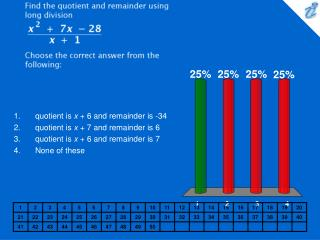DownloadDownload PresentationFind the quotient and remainder using long division {image} Choose the correct answer from the following:

# Find the quotient and remainder using long division {image} Choose the correct answer from the following:

Télécharger la présentation## Find the quotient and remainder using long division {image} Choose the correct answer from the following:

- - - - - - - - - - - - - - - - - - - - - - - - - - - E N D - - - - - - - - - - - - - - - - - - - - - - - - - - -
##### Presentation Transcript

1. Find the quotient and remainder using long division {image} Choose the correct answer from the following: • quotient is x + 6 and remainder is -34 • quotient is x + 7 and remainder is 6 • quotient is x + 6 and remainder is 7 • None of these

2. Find the quotient and remainder using synthetic division {image} Choose the correct answer from the following. • the quotient is x2 + 1x + 8 and the remainder is 6 • the quotient is 6 and the remainder is x2 + 1x + 8 • the quotient is x 2 + 1x - 8 and the remainder is 6 • the quotient is 6 and the remainder is x2 - 1x + 8 • None of these

3. Find the quotient and remainder using synthetic division. {image} Choose the correct answer from the following: • the quotient is x2 + 3x + 9 and the remainder is 0 • the quotient is x2 - 3x + 9 and the remainder is 0 • the quotient is x2 - 9 and the remainder is 3 • None of these

4. Use synthetic division and the remainder theorem to evaluate P ( -2 ), for P ( x ) = -4 x 6 + 9 x 5 + 40 x 4 - 5 x 2 + 12 x + 128 Choose the correct answer from the following. • 180 • 181 • 190 • 280 • 80 • None of these

5. Given x = 5 is zero of P (x) = x3 - 7 x2 + 2 x + 40, find all other zeros of P (x). Choose the correct answer from the following. • x = 4, x = -2 • x = -4, x = -2 • x = -4, x = 2 • x = 4, x = 2 • None of these